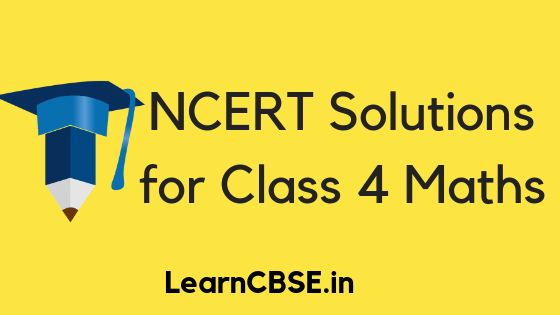NCERT Solutions for Class 4 Maths Math Magic includes all the questions given in the CBSE class 4 maths textbook. All solutions contain a detailed explanation so that it is easy to follow for the young student. Free NCERT solutions for class 4 math, consists of solutions for all the questions organised in a chapter-wise manner. The solutions provided here are with respect to the NCERT curriculum.

## NCERT Solutions for Class 4 Maths Math Magic

NCERT Solutions for Class 4 Maths Math Magic Workbook Pdf, Worksheets, Notes, Questions and Answers.

NCERT Solutions for Class 4

Math is a very important subject and one that a lot of students struggle with. As elementary school is when they learn the basics, it is integral to their academic growth that they lay down a strong foundation.

Hence, here we provide easy to access CBSE 4th class maths ncert textbook solutions, organised in a chapter-wise, question-wise manner so that they can easily find out what they’re looking for.

### Class 4 Maths Chapter Building with Bricks

In this chapter, the students learn to make patterns with bricks. They come to understand the size and shape of bricks and learn to identify the different patterns formed by them.

### Class 4 Maths Chapter 2 Long and Short

In this chapter, students learn to deal with lengths. They learn about measurement in centimetres and meters, and of conversion between the same. They are taught this important concept in a simple way using dot to dot distances.

### Class 4 Maths Chapter 3 A trip to Bhopal

This is a very exciting and interesting chapter for the students. They are introduced to everyday math through a short adventure in Bhopal.

### Class 4 Maths Chapter 4 Tick-tick-tick

This is one of the more important chapters in the syllabus. In this chapter, students learn to tell the time from analogue clocks. As they grow, babies develop a somewhat vague sense of time and understand how day transitions into night. This chapter will help them understand it better and will inculcate in them the value of punctuality.

### Class 4 Maths Chapter 5 The Way The World Looks

This chapter helps students appreciate dimensions and perspectives in the real world. They learn to intuitively differentiate between 3-D and 2-D objects, and understand how objects appear in different viewing profiles.

### Class 4 Maths Chapter 6 The Junk Seller

This chapter introduces students to the concept of buying and selling through a story format. They begin to grasp the idea of earning, expenditure, sales and loan. This in a way sets up the students to function better in the real world.

### Class 4 Maths Chapter 7 Jugs and Mugs

This chapter focusses on units of measurement of liquids. The students are introduced to this concept by measuring water. They begin from large units like litre and move on to smaller ones like millilitre. They are also taught how to convert between the two.

### Class 4 Maths Chapter 8 Carts and Wheels

Circles are the most difficult geometric shape for a student to master, as it does not have proper sides of fixed lengths. Hence, this chapter makes them comfortable with the idea of circles and radii. They also get an intuitive feel for the perimeter of a circle.

### Class 4 Maths Chapter 9 Halves and Quarters

Fractions are a difficult concept to master as until now, the students have learnt to deal only with whole numbers. So, this chapter introduces them to the concept gradually, using chapatis and chocolates. They understand about wholes, halves and quarters.

### Class 4 Maths Chapter 10 Play with Patterns

The NCERT syllabus ensures that students do not rote learn but instead learn to appreciate the subject. This chapter is one such example, as it introduces students to the concept of patterns. It develops their thinking and analysing skill as they learn to identify patterns in a string of characters.

### Class 4 Maths Chapter 11 Tables and Shares

This chapter teaches students the applications of division in everyday life. They learn to divide objects into rows and groups.

### Class 4 Maths Chapter 12 How Heavy? How Light?

This chapter teaches students the concept of weight measurement. They learn to use the various units of the same. They learn the practical application of weights in balances and learn how to use different loads to match up to a given weight.

### Class 4 Maths Chapter 13 Fields and Fences

This chapter introduces students to the idea of area and perimeter by talking about more understandable things, like fields and fences.

### Class 4 Maths Chapter 14 Smart Charts

This chapter introduces students to the very crucial concept of handling pictorial information. Data presented in pictorial forms are easier to analyse. Hence, the students are provided with the tools to work with pie charts and pictographs. This also helps them develop a keener intuition for numbers.

We have tried to provide clear, accurate NCERT Solutions for Class 4 Maths Math Magic for all the questions, as these are important concepts that may not be easy to master in the first try. To better understand this material, you might want to clear up your 3rd grade math concepts.